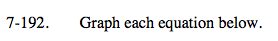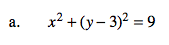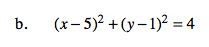Home > A2C > Chapter 7 > Lesson 7.3.3 > Problem7-192

7-192.
1. Graph each equation below. Homework Help ✎

1. x2 + (y − 3)2 = 9

2. (x − 5)2 + (y − 1)2 = 4What kind of equation does this look like?

This is the graphingform of a circle equation.
center: (0,3)